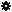﻿ Object Model: NurbsCurve.GetNormalizedUFromU

NurbsCurve.GetNormalizedUFromU

## NurbsCurve.GetNormalizedUFromU### Description

Returns the normalized equivalent of U value.

### C# Syntax

 ```Double NurbsCurve.GetNormalizedUFromU( Double in_dUValue ); ```

### Scripting Syntax

 ```oDouble = NurbsCurve.GetNormalizedUFromU( UValue ); ```

### Parameters

Parameter Type Description
UValue Double The UValue from which we want the Normalized equivalent.

### Examples

#### VBScript Example

 ```set oRoot = Application.ActiveProject.ActiveScene.Root set oArc = oRoot.AddGeometry( "Arc", "NurbsCurve" ) UNormalized = oArc.ActivePrimitive.Geometry.Curves(0).GetNormalizedUFromU( 3.0 ) LogMessage "The normalized equivalent of 3.0 is U :" & UNormalized ```